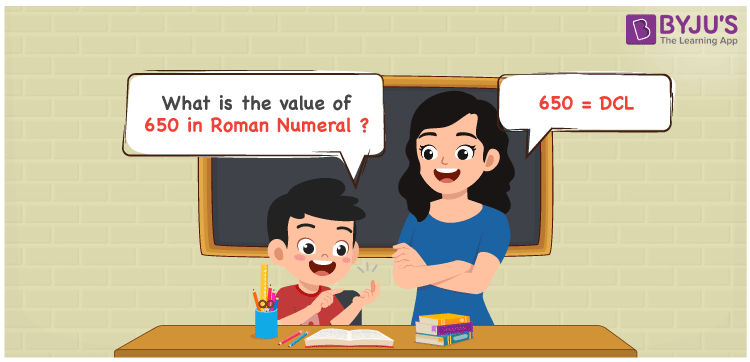# 650 in Roman Numerals

650 in Roman Numerals is DCL. Any Roman numeral uses only the 7 primary symbols which are I, V, X, L, C, D, and M. Here D represents 500, C represents 100 and L represents 50. By following the additive property of Roman Numerals, 650 is represented as DCL. What are Roman numerals? can be referred for more details. The details on how to write 650 is given in the next section of this article.

 Number Roman Numeral 650 DCL

## How to Write 650 in Roman Numerals?To convert 650 in Roman Numerals, we need to represent 650 as the sum of the fundamental symbols. 650 can be broken down as the sum of 600 and 50. But we do not have a primary symbol for 600. Hence it needs to be represented as a sum of 100 and 500.. And L is the symbol for 50. So 650 will be 500 + 100 + 50.

650 = 500 + 100 + 50

650 = D + C + L

650 = DCL

## Video Lesson on Roman Numerals## Frequently Asked Questions on 650 in Roman Numerals

Q1

### How to write the number 650 in Roman Numerals?

650 in Roman Numerals is written as DCL.
Q2

### Does DCL represent Roman Numerals?

Yes. DCL represents Roman Numerals and it is the number 650
Q3

### How to represent DCL in the Hindu-Arabic number system?

The Roman Numerals DCL is represented as 650 in the Hindu-Arabic number system.